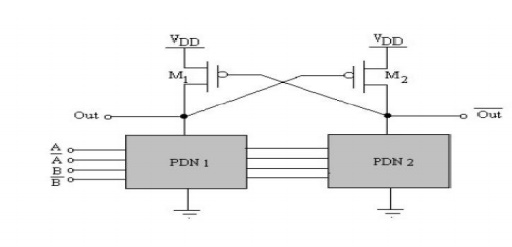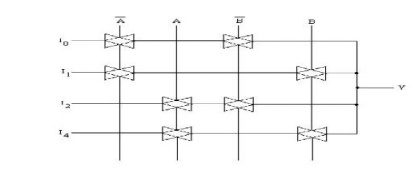Home | | VLSI Design | Important Short Questions and Answers: Combinational and Sequential Circuit Design

# Important Short Questions and Answers: Combinational and Sequential Circuit Design

VLSI Design - Combinational and Sequential Circuit Design - Important Short Questions and Answers: Combinational and Sequential Circuit Design

1. What are the static properties of complementary CMOS Gates?

a.        They exhibit rails-to-rail swing with VOH = VDD and VOL = GND.

b.        The circuits have no static power dissipation, since the circuits are designed such that the pull-down and pull-up networks are mutually exclusive.

c.         The analysis of the DC voltage transfer characteristics and the noise margins is more complicated than for the inverter, as these parameters depend upon the data input patterns applied to the gate.

2. Draw the equivalent RC model for a two-input NAND gate.3. What are the major limitations associated with complementary CMOS gate? a. The number of transistors required to implement an N fan-in gate is 2N. This can result in a significantly large implementation area.

b. The propagation delay of a complementary CMOS gate deteriorates rapidly as a function of the fan-in.

4. What is meant by ratioed logic?

In ratioed logic, a gate consists of an nMOS pull-down network that realizes the logic function and a simple load device, which replace the entire pull-up network. A ratioed logic which uses a grounded pMOS load is referred to as a pseudo-nMOS gate

5. What is true single phase clocked register?

The True single-phase clocked register (TSPCR) uses a single clock, CLK. For the positive latch, when CLK is high, the latch is in the transparent mode and corresponds to two cascaded inverters; the latch is non-inverting, and propagates the input to the output. On the other hand, when CLK=0, both inverters are disabled, and the latch is in the hold mode.

6. Define a tally circuit.

A tally circuit counts the number of inputs that are high and outputs the answer. If there are N inputs there are N +1 possible outputs, corresponding to 0, 1, 2, …. N inputs that are high.

7. Give the NAND-GATE8. Give the 3 INPUT NAND-GATE9. Draw the CMOS implementation of 4-to-1 MUX using transmission gates .10.   What are the various mo deling used in Verilog?

1. Gate-level modeling

2. Data-flow modeling

3. Switch-level modeling

4. Behavioral modeling

11.   What is the structural ga te-level modeling?

Structural modeling deescribes a digital logic networks in terms of t he components that make up the system. Gate-lev el modeling is based on using primitive logic gates and specifying how they are wired together.

12.What is Switch-level mod eling?

Verilog allows switch-level modeling that is based on the behavior of MOSFETs. Digital circuits at the MOS-transistor level are described using the MOSFET switches.

13.   What are the types of programmable device?

Programmable logic structure

Programmable Interconnect

Reprogrammable gate arry

14.   What is CLB?

CLB means Configurable Log ic Block.

15.What are the two types of MOSFET?

Two types of MOSET are n -channel MOSET and p-channel MOSFET. These are known as n-MOS and p-MOS.

16.which MOS can pass logic 1 and logic 0 strongly?

p-MOS can pass strong logic 1

n-MOS can pass strong logic 0

17. What is AOI logic function?

AND OR Invert logic funct ion (AOI) implements operation in the or der of AND, OR, NOT operations. So this logic function is known as AOI logic function.

18. What is bubble pushing?

According to De Morgan’s la ws,So NAND gate may be draw n as bubbled OR gate. Bubbles are introduce d in the input side. This concept is known as bubble pushing.20. What is OAI 221 Gate?

OAI 221, here 221 refers to n umber of inputs in each section.

21.   Write the features of CM OS Domino Logic?

ü  These structures occup y small area compared with conventional lo gic structure.

ü  Parasitic capacitance is to be small to increase the speed.

ü  Each gate can make o ne ‘logic 1’ to ‘logic 0’ transition.

22.   What are the tally circuits?

ü  Tally circuits one of th e applications of the pass transistor logic.

ü  It is used to count the number of inputs which are high and the outp ut is produced.

23.   What are the various for ms of inverter based CMOS logic?

i.          Pseudo N-MOS logic

ii.        Dynamic C-MOS logic

iii.     Clocked C-MOS logic

iv.     C-MOS domino logic

v.        n-p C-MOS logic

24.   What is PIP in XILINIX?

PIP means Programmable Int erconnect Point in XILINIX.

ü  Simplicity

ü  Small size